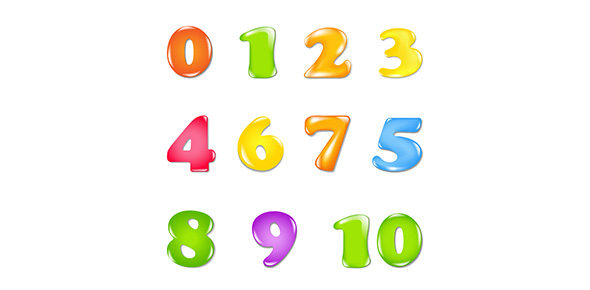# Unit 1 Preassessment: Rational Number Uses

18 Questions | Total Attempts: 166SettingsThis quiz will not serve as a grade, but as a means to determine areas of strength and weakness. Please take your time and answer each question to the best of your ability.

• 1.
Which ratio describes 20 basset hounds out of 45 dogs as a fraction in simplest form?
• A.

20/45

• B.

9/4

• C.

9:4

• D.

4/9

• 2.
Bonnie purchased a 30-ounce package of Chocolate Chunk Cookies for \$2.40.  What is the cost per ounce?
• A.

\$0.88 per oz.

• B.

\$8.00 per oz.

• C.

\$0.80 per oz.

• D.

\$0.08 per oz.

• 3.
Bus Number 712 traveled 150 miles on 10 gallons of gas.  How many miles per gallon does the bus get?
• A.

15

• B.

150

• C.

1.5

• D.

0.15

• 4.
Approximately 105 out of 350 teens have their own savings account.  Express the ratio 105:350 as a fraction in simplest form.
• A.

3/1

• B.

1/3

• C.

10/3

• D.

3/10

• 5.
Express the ratio that compares the number of purple Skittles to the total number of Skittles.
• A.

2/23

• B.

3/15

• C.

1/4

• D.

2/3

• 6.
Express 0.4 as a fraction in simplest form.
• A.

2/5

• B.

4/100

• C.

1/4

• D.

4/10

• 7.
The White House is approximately 18.05 acres.  Express this decimal as a mixed number in simplest form.
• A.

18 and 1/5

• B.

18 and 5/100

• C.

18 and 1/50

• D.

18 and 1/20

• 8.
Express 0.6 as a fraction in simplest form.
• A.

6/100

• B.

3/5

• C.

6/10

• D.

1/2

• 9.
Write two and twelve hundredths as a mixed number in simplest form.
• A.

2 and 6/40

• B.

2 and 6/50

• C.

2 and 3/25

• D.

2

• 10.
Write 7 and  4/5 as a decimal.
• A.

7.8

• B.

7.9

• C.

7.4

• D.

7.45

• 11.
Replace the # with a number so that the fractions are equivalent:  18/21 = 6/#
• A.

7

• B.

3

• C.

4

• D.

6

• 12.
Write 5/20 in simplest form.
• A.

1/5

• B.

1/4

• C.

4/5

• D.

1/20

• 13.
Write 42/54 in simplest form.
• A.

8/10

• B.

7/9

• C.

8/9

• D.

21/27

• 14.
State the least common denominator of 8/15 and 13/25.
• A.

100

• B.

300

• C.

25

• D.

75

• 15.
Write 1/4, 1/3, and 2/7 in order from least to greatest.
• A.

2/7, 1/3, 1/4

• B.

1/3, 1/4, 2/7

• C.

1/4, 2/7, 1/3

• D.

2/7, 1/4, 1/3

• 16.
Write 6x6x6x6x6 using exponents.
• A.

6^6

• B.

1^6

• C.

6^5

• D.

6^4

• 17.
Evaluate 4^5  ("Four to the fifth power").
• A.

1024

• B.

256

• C.

64

• D.

625

• 18.
Which number is a perfect square?
• A.

36

• B.

26

• C.

32

• D.

11

Related TopicsBack to top